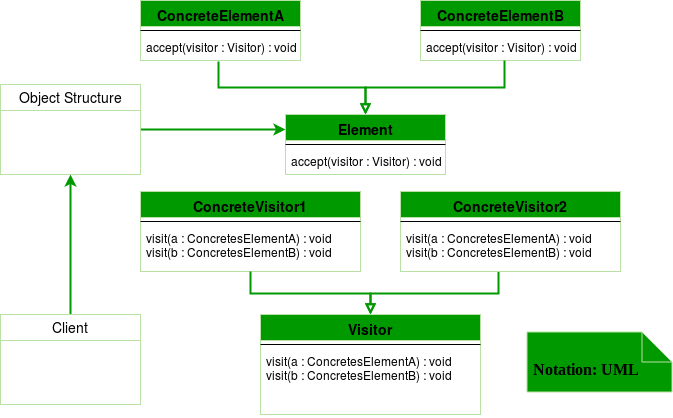# Visitor design pattern

• Difficulty Level : Hard
• Last Updated : 12 Mar, 2019

Visitor design pattern is one of the behavioral design patterns. It is used when we have to perform an operation on a group of similar kind of Objects. With the help of visitor pattern, we can move the operational logic from the objects to another class.

The visitor pattern consists of two parts:

• a method called Visit() which is implemented by the visitor and is called for every element in the data structure
• visitable classes providing Accept() methods that accept a visitor

UML Diagram Visitor design patternDesign components

• Client : The Client class is a consumer of the classes of the visitor design pattern. It has access to the data structure objects and can instruct them to accept a Visitor to perform the appropriate processing.
• Visitor : This is an interface or an abstract class used to declare the visit operations for all the types of visitable classes.
• ConcreteVisitor : For each type of visitor all the visit methods, declared in abstract visitor, must be implemented. Each Visitor will be responsible for different operations.
• Visitable : This is an interface which declares the accept operation. This is the entry point which enables an object to be “visited” by the visitor object.
• ConcreteVisitable : These classes implement the Visitable interface or class and defines the accept operation. The visitor object is passed to this object using the accept operation.

Let’s see an example of Visitor design pattern in Java.

 `interface` `ItemElement``{``    ``public` `int` `accept(ShoppingCartVisitor visitor);``}`` ` `class` `Book ``implements` `ItemElement``{``    ``private` `int` `price;``    ``private` `String isbnNumber;``  ` `    ``public` `Book(``int` `cost, String isbn)``    ``{``        ``this``.price=cost;``        ``this``.isbnNumber=isbn;``    ``}``  ` `    ``public` `int` `getPrice() ``    ``{``        ``return` `price;``    ``}``  ` `    ``public` `String getIsbnNumber() ``    ``{``        ``return` `isbnNumber;``    ``}``  ` `    ``@Override``    ``public` `int` `accept(ShoppingCartVisitor visitor) ``    ``{``        ``return` `visitor.visit(``this``);``    ``}``  ` `}`` ` `class` `Fruit ``implements` `ItemElement ``{``    ``private` `int` `pricePerKg;``    ``private` `int` `weight;``    ``private` `String name;``  ` `    ``public` `Fruit(``int` `priceKg, ``int` `wt, String nm)``    ``{``        ``this``.pricePerKg=priceKg;``        ``this``.weight=wt;``        ``this``.name = nm;``    ``}``  ` `    ``public` `int` `getPricePerKg() ``    ``{``        ``return` `pricePerKg;``    ``}``  ` `    ``public` `int` `getWeight() ``    ``{``        ``return` `weight;``    ``}``  ` `    ``public` `String getName()``    ``{``        ``return` `this``.name;``    ``}``  ` `    ``@Override``    ``public` `int` `accept(ShoppingCartVisitor visitor) ``    ``{``        ``return` `visitor.visit(``this``);``    ``}``  ` `}`` ` `interface` `ShoppingCartVisitor ``{``  ` `    ``int` `visit(Book book);``    ``int` `visit(Fruit fruit);``}`` ` `class` `ShoppingCartVisitorImpl ``implements` `ShoppingCartVisitor``{``  ` `    ``@Override``    ``public` `int` `visit(Book book)``    ``{``        ``int` `cost=``0``;``        ``//apply 5\$ discount if book price is greater than 50``        ``if``(book.getPrice() > ``50``)``        ``{``            ``cost = book.getPrice()-``5``;``        ``}``        ``else` `            ``cost = book.getPrice();``             ` `        ``System.out.println(``"Book ISBN::"``+book.getIsbnNumber() + ``" cost ="``+cost);``        ``return` `cost;``    ``}``  ` `    ``@Override``    ``public` `int` `visit(Fruit fruit) ``    ``{``        ``int` `cost = fruit.getPricePerKg()*fruit.getWeight();``        ``System.out.println(fruit.getName() + ``" cost = "``+cost);``        ``return` `cost;``    ``}``  ` `}`` ` `class` `ShoppingCartClient ``{``  ` `    ``public` `static` `void` `main(String[] args) ``    ``{``        ``ItemElement[] items = ``new` `ItemElement[]{``new` `Book(``20``, ``"1234"``),``                              ``new` `Book(``100``, ``"5678"``), ``new` `Fruit(``10``, ``2``, ``"Banana"``),``                              ``new` `Fruit(``5``, ``5``, ``"Apple"``)};``  ` `        ``int` `total = calculatePrice(items);``        ``System.out.println(``"Total Cost = "``+total);``    ``}``  ` `    ``private` `static` `int` `calculatePrice(ItemElement[] items) ``    ``{``        ``ShoppingCartVisitor visitor = ``new` `ShoppingCartVisitorImpl();``        ``int` `sum=``0``;``        ``for``(ItemElement item : items)``        ``{``            ``sum = sum + item.accept(visitor);``        ``}``        ``return` `sum;``    ``}``  ` `}`

Output:

```Book ISBN::1234 cost =20
Book ISBN::5678 cost =95
Banana cost = 20
Apple cost = 25
Total Cost = 160
```

Here, in the implementation if accept() method in all the items are same but it can be different. For example there can be logic to check if item is free then don’t call the visit() method at all.

• If the logic of operation changes, then we need to make change only in the visitor implementation rather than doing it in all the item classes.
• Adding a new item to the system is easy, it will require change only in visitor interface and implementation and existing item classes will not be affected.

• We should know the return type of visit() methods at the time of designing otherwise we will have to change the interface and all of its implementations.
• If there are too many implementations of visitor interface, it makes it hard to extend.

Another example of visitor pattern in C++

 `//Write CPP code here`` ` `#include ``using` `namespace` `std;`` ` `class` `Stock``{``  ``public``:``    ``virtual` `void` `accept(``class` `Visitor *) = 0;``     ` `};`` ` `class` `Apple : ``public` `Stock``{``  ``public``:``    ``/*virtual*/` `void` `accept(Visitor *);``    ``void` `buy()``    ``{``        ``cout << ``"Apple::buy\n"``;``    ``}``    ``void` `sell()``    ``{``        ``cout << ``"Apple::sell\n"``;``    ``}``     ` `};``class` `Google : ``public` `Stock``{``  ``public``:``    ``/*virtual*/` `void` `accept(Visitor *);``    ``void` `buy()``    ``{``        ``cout << ``"Google::buy\n"``;``    ``}`` ` `    ``void` `sell()``    ``{``        ``cout << ``"Google::sell\n"``;``    ``}``};`` ` `class` `Visitor``{``  ``public``:``    ``virtual` `void` `visit(Apple *) = 0;``    ``virtual` `void` `visit(Google *) = 0;``    ``//private:``    ``static` `int` `m_num_apple, m_num_google;``    ``void` `total_stocks()``    ``{``        ``cout << ``"m_num_apple "` `<< m_num_apple ``             ``<< ``", m_num_google "` `<< m_num_google << ``'\n'``;``    ``}``};``int` `Visitor::m_num_apple = 0;``int` `Visitor::m_num_google = 0;``class` `BuyVisitor : ``public` `Visitor``{``  ``public``:``    ``BuyVisitor()``    ``{``        ``m_num_apple = m_num_google = 0;``    ``}``    ``/*virtual*/` `void` `visit(Apple *r)``    ``{``        ``++m_num_apple;``        ``r->buy();``        ``cout << ``"m_num_apple "` `<< m_num_apple << endl;``    ``}``    ``/*virtual*/` `void` `visit(Google *b)``    ``{``        ``++m_num_google;``        ``b->buy();``        ``cout << ``" m_num_google "` `<< m_num_google << ``'\n'``;``    ``}``};`` ` `class` `SellVisitor : ``public` `Visitor``{``  ``public``:``    ``/*virtual*/` `void` `visit(Apple *a)``    ``{``         ` `        ``--m_num_apple;``        ``a->sell();``        ``cout << ``"m_num_apple "` `<< m_num_apple << endl;``    ``}``    ``/*virtual*/` `void` `visit(Google *g)``    ``{``        ``--m_num_google;``        ``g->sell();``        ``cout << ``"m_num_google "` `<< m_num_google << endl;``    ``}``};`` ` `void` `Apple::accept(Visitor *v)``{``    ``v->visit(``this``);``}`` ` `void` `Google::accept(Visitor *v)``{``    ``v->visit(``this``);``}`` ` `int` `main()``{``    ``Stock *set[] = { ``new` `Apple, ``new` `Google, ``new` `Google,``                     ``new` `Apple, ``new` `Apple, 0 };`` ` `    ``BuyVisitor buy_operation;``    ``SellVisitor sell_operation;``    ``for` `(``int` `i = 0; set[i]; i++)``    ``{``        ``set[i]->accept(&buy_operation);``    ``}``    ``buy_operation.total_stocks();`` ` `    ``for` `(``int` `i = 0; set[i]; i++)``    ``{`` ` `        ``set[i]->accept(&sell_operation);``    ``}``    ``sell_operation.total_stocks();``}`

Output:

```Apple::buy
m_num_apple 1
m_num_apple 2
m_num_apple 3
m_num_apple 3, m_num_google 2
Apple::sell
m_num_apple 2
Apple::sell
m_num_apple 1
Apple::sell
m_num_apple 0
m_num_apple 0, m_num_google 0
```

This article is contributed by Saket Kumar. If you like GeeksforGeeks and would like to contribute, you can also write an article using contribute.geeksforgeeks.org or mail your article to contribute@geeksforgeeks.org. See your article appearing on the GeeksforGeeks main page and help other Geeks.

Please write comments if you find anything incorrect, or you want to share more information about the topic discussed above.

My Personal Notes arrow_drop_up